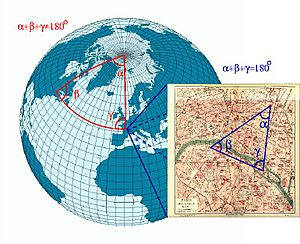# Manifold facts for kids

Kids Encyclopedia FactsOn a sphere, the sum of the angles of a triangle is not equal to 180°. A sphere is not a Euclidean space, but locally the laws of the Euclidean geometry are good approximations. In a small triangle on the face of the earth, the sum of the angles is almost 180°. A sphere can be represented by several two dimensional maps, therefore a sphere is a manifold.

A manifold is a concept from mathematics. Making a manifold is like making a flat map of a sphere (the Earth).

The Earth is a sphere, a three dimensional object of geometry. Yet, maps (two-dimensional representations) can be made of the Earth. At the edges of a certain map, the map needs to be changed. That way it is possible to make a two dimensional image of the whole surface of the Earth. There need to be rules, on how to change the maps, and some areas (near the edges of the map) will be on more than one map. It is not possible to make one map only, which would have no edges. This map would either have edges (and overlapping areas), or there would be some places where the paper was torn. Tearing and overlapping isn't allowed.

Each manifold has a dimension. This is the dimension of the maps, in the example above. It is the same for all maps.

## Images for kidsManifold Facts for Kids. Kiddle Encyclopedia.